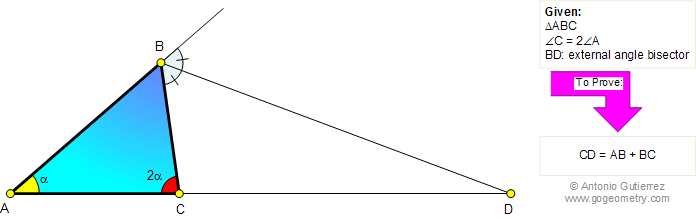# Online Geometry Problem 739: Triangle, Double Angle, External Angle Bisector. Congruence Level: High School, Honors Geometry, College, Mathematics Education

 The figure shows a triangle ABC so that the angle C is double of angle A. If BD is the external angle bisector of angle B, prove that CD = AB + BC.Home | Search | Geometry | Problems | All Problems | Open Problems | Visual Index | 731-740 | Triangles | Isosceles Triangle | Angle Bisector | Double Angle | Email | Solution / comment | By Antonio Gutierrez